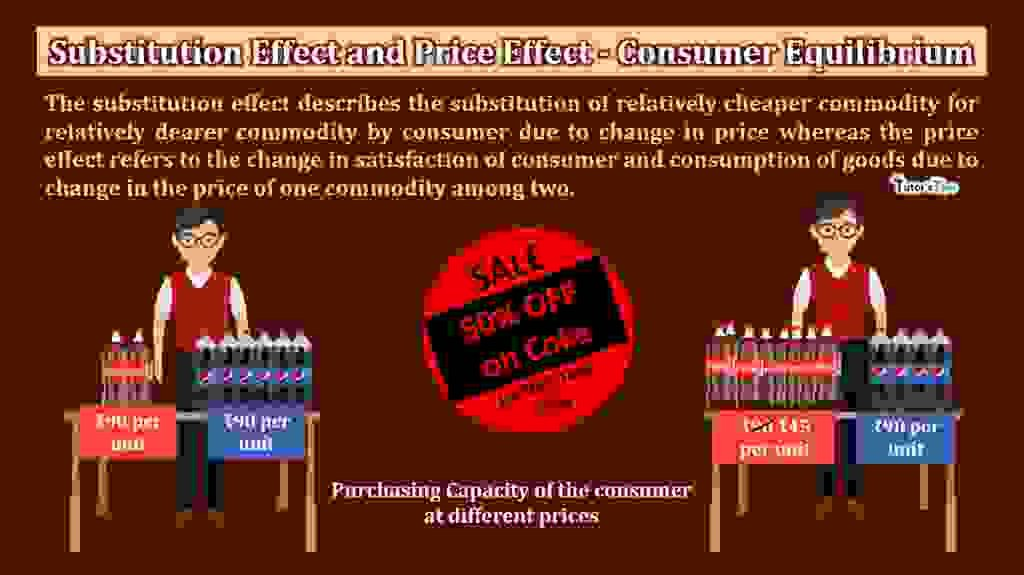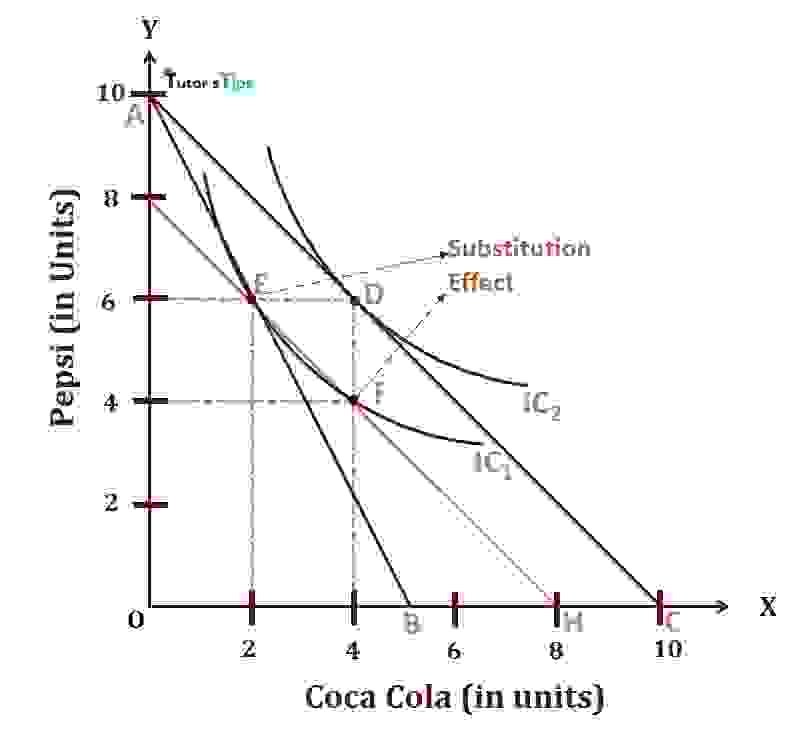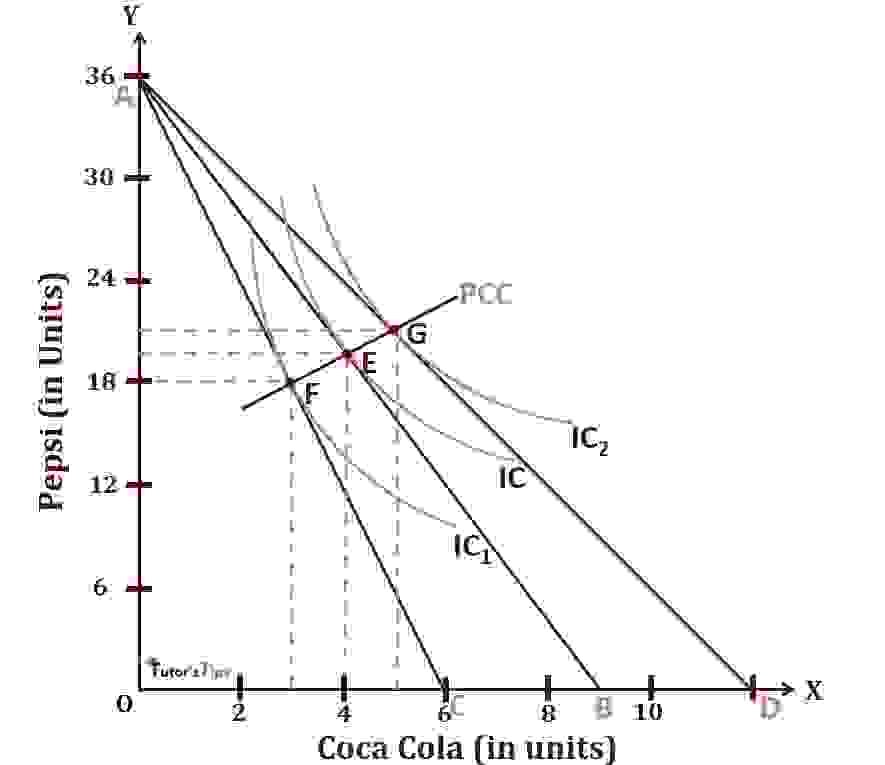# Substitution Effect and Price Effect – Consumer EquilibriumSubstitution effect and the price effect influences the consumer Equilibrium. The substitution effect describes the change in quantity purchased due to the change in the price of a commodity. Whereas the price effect refers to the change in consumption of goods due to a change in the price of one commodity among two. The income is assumed to be constant for both effects.

The Content covered in this article:

## Substitution Effect :

Substitution effect refers to the change in quantity demanded of a commodity by a consumer due to change in its relative price, while the income of consumer remains the same.

If with the change in prices of commodities, the income of the consumer changes in such a way that his real income remains the same, then the consumer will switch to substitute goods at cheaper prices. Consequently, It will affect the purchasing pattern of the consumer, and this effect is known as the Substitution Effect.

### Illustration:

Suppose the income of the consumer is Rs.200, which he spends on the purchase of Pepsi and Coca Cola. Assuming, the price of Pepsi as Rs.20 and price of Coca Cola as Rs.40, the consumer is able to buy 6 units of Pepsi and 2 units of Coca Cola and finds himself in equilibrium.

When the price of Coca Cola falls to Rs.20, and the price of Pepsi remaining the same, the consumer can buy the same quantity of both commodities at just Rs.160. It means, he is left with Rs.40 after these purchases. In other words, his real income has gone up.

If we take these 40 Rs. from him, his real income will remain the same. It implies, he will continue his purchase at Rs.160 only with the same quantity. But, as the relative price of Coca Cola is less, a rational consumer would like to switch from Pepsi to Coca Cola. He may buy 4 units of Pepsi and 4 units of Coca Cola with this income of Rs.160. This substitution made by the consumer of a relatively cheaper commodity(Coca Cola) for a relatively dearer commodity(Pepsi) is called the Substitution effect.

Thus, it is evident from the above example that substitution effect means those changes in the quantities of goods bought which arise due to change in their relative prices while the real income of the consumer remains constant. As a result of the substitution effect, the consumer’s satisfaction neither increases nor decreases, his level of satisfaction remains the same. In other words, his indifference curve remains the same depicting, same level of satisfaction.

### Graphical Representation of the Substitution Effect:Substitution Effect on Consumer Equilibrium

In fig, the quantities purchased of Coca Cola and Pepsi are shown on X-axis and Y-axis respectively. AB is the original budget line with an income level of Rs.200 and IC1 is the original indifference curve. Here, the consumer is equilibrium at point E, by getting 6 units of Pepsi and 2 units of Coca-Cola. As the price of Coca Cola falls, the AB budget line shifts outward to the right as AC and tangent to the higher indifference curve IC2 at point D which will be the new equilibrium point. Here, the consumer is able to buy more of both commodities and the real income will be more than before.

To make the real income of consumer constant as before, we will take away his extra income i.e. Rs.40. When this money has been taken away, GH will be the new budget line with the income level of Rs.160. The new budget line is tangent to indifference curve IC1 at F, which is the new equilibrium point of the consumer with constant real income.

It indicates:

1. The consumer remains at same old indifference curve IC1 and his level of satisfaction remains the same.
2. The consumer will substitute the relative cheaper commodity i.e. Coca Cola for relatively dearer commodity i.e. Pepsi. He will substitute 2 units of Coca Cola for 2 units of Pepsi.

This substitution of the relatively cheaper commodity for the relatively dearer commodity by the consumer is known as the Substitution Effect. In this case, the movement of equilibrium point from E to F on the same indifference curve IC1 is known as the Substitution Effect.

## Price Effect :

Price Effect refers to the change in the consumption of the commodities when the price of one of the commodity changes, provided the price of other commodity and income of consumer being the same.

In the words of Lipsey,

“The price effect shows how much satisfaction of the consumer varies due to the change in the consumption of two goods as the price of one changes the price of the other and the money income remains the constant.”

### Graphical Representation of Price Effect:Price Effect on Consumer Equilibrium

In fig, the consumption of Coca Cola and Pepsi is shown on X-axis and Y-axis. AB is the original budget line with an income level of Rs 360 and IC is the original indifference curve showing equilibrium point at E. It is assumed that the income of consumer and price of Pepsi is constant and the price of Coca Cola fluctuates.

When the price of Coca Cola rises from Rs.40 to Rs.60, then new budget line AC which touches the lower indifference curve at a point F, the new equilibrium point. It means a rise in the price of any commodity will decrease the satisfaction of the consumer. In other words, demand for Coca Cola decreases from 4 units to 3 units and the demand for Pepsi will decrease from 20 units to 18 units. Here, the price effect on Coca Cola is (5-4)=1unit and on Pepsi, it is (20-18)=1 unit.

On the contrary, if the price of Coca Cola falls from Rs.40 to Rs.30, then the new budget line AD will touch the higher indifference curve at point G, the new equilibrium point. It means a fall in the price of any commodity will increase the satisfaction of the consumer. By joining together different equilibrium points E, F and G, we get Price Consumption Curve(PCC).

Thanks Please share with your friends

Comment if you have any question.

References:

Introductory Microeconomics – Class 11 – CBSE (2020-21)